### ATLAS_2016_I1426515

Back to index

The production of $W$ boson pairs in proton-proton collisions at $\sqrt{s} = 8$ TeV is studied using data corresponding to 20.3 fb${}^{-1}$ of integrated luminosity collected by the ATLAS detector during 2012 at the CERN Large Hadron Collider. The $W$ bosons are reconstructed using their leptonic decays into electrons or muons and neutrinos. Events with reconstructed jets are not included in the candidate event sample. A total of 6636 $WW$ candidate events are observed. Measurements are performed in fiducial regions closely approximating the detector acceptance. The integrated measurement is corrected for all acceptance effects and for the $W$ branching fractions to leptons in order to obtain the total $WW$ production cross section, which is found to be $71.1 \pm 1.1$ (stat) ${}^{+5.7}_{-5.0}$ (syst) $\pm 1.4$ (lumi) pb. This agrees with the next-to-next-to-leading-order Standard Model prediction of $63.2 {}^{+1.6}_{-1.4}$ (scale) $\pm 1.2$ (PDF) pb. Fiducial differential cross sections are measured as a function of each of six kinematic variables. The distribution of the transverse momentum of the leading lepton is used to set limits on anomalous triple-gauge-boson couplings.

ATLAS_2016_I1426515_d01-x01-y01: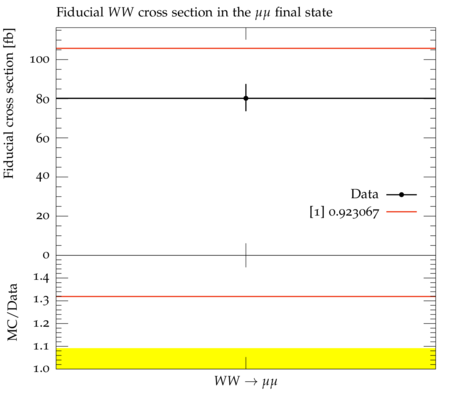ATLAS_2016_I1426515_d02-x01-y01: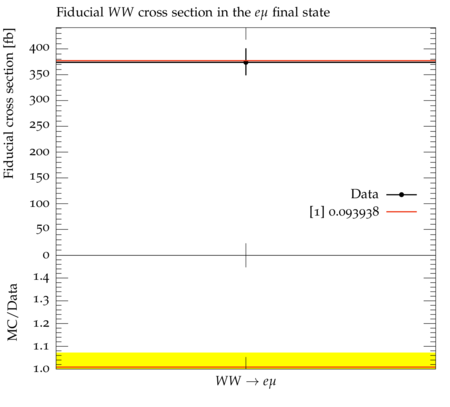ATLAS_2016_I1426515_d10-x01-y01: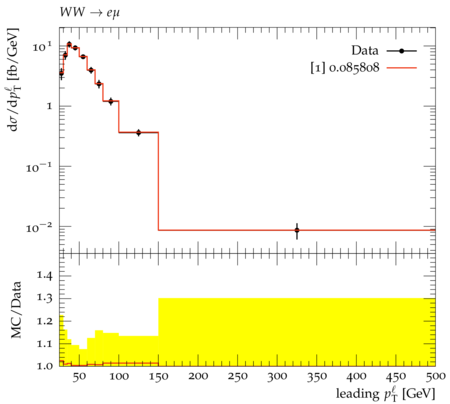ATLAS_2016_I1426515_d11-x01-y01: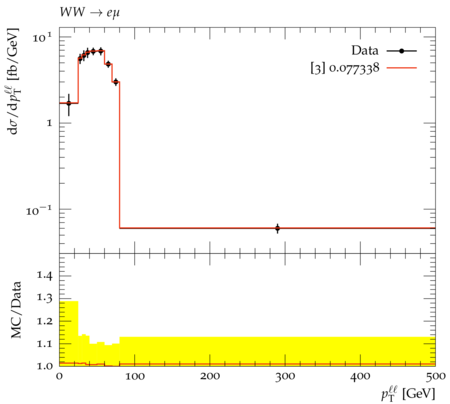ATLAS_2016_I1426515_d12-x01-y01: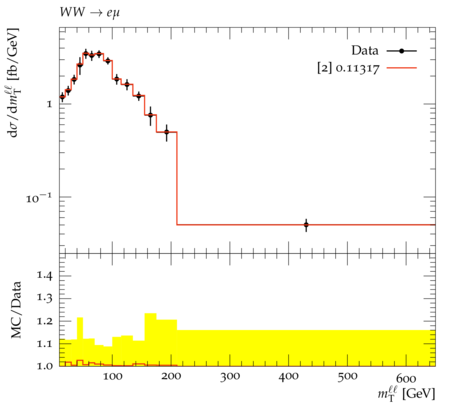ATLAS_2016_I1426515_d13-x01-y01: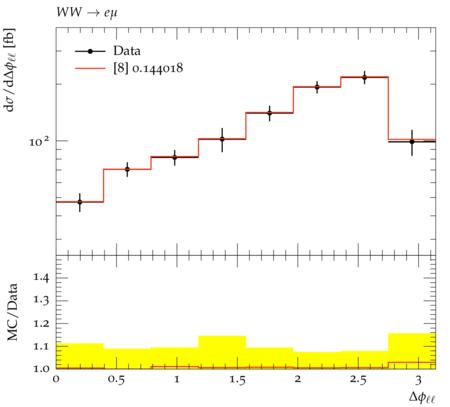ATLAS_2016_I1426515_d14-x01-y01: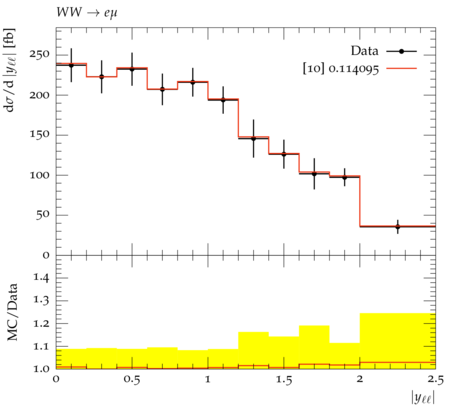ATLAS_2016_I1426515_d15-x01-y01: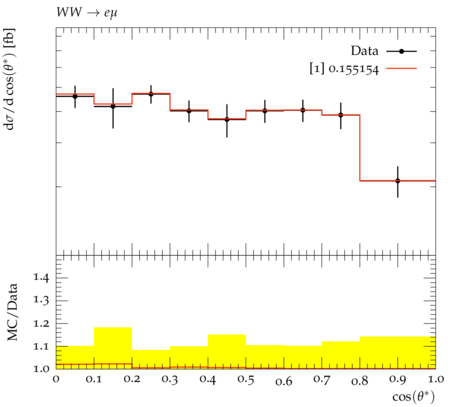Generated at Monday, 18. September 2017 09:14PM